Next: The survey of ISB Up: ISB corrections to the Previous: Nearly degenerate -orbitals

## Theoretical uncertainties and error analysis

Based on the discussion presented in Secs. 3.1 and 3.2, the recommended calculated values of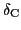for the superallowed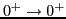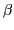-decay are determined by averaging over three relative orientations of shapes and currents. Only in the case of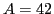, we adopt foran arithmetic mean over the four configurations associated with different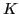-orbitals.

To minimize uncertainties in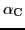andassociated with the truncation of HO basis in HFODD, we used different HO spaces in different mass regions, cf. Sec. 2.3. With this choice, the resulting systematic errors due the basis cut-off should not exceed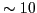%. To illustrate the dependence ofon the number of HO shells, Fig. 5 shows the case of the superallowed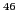V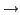Ti transition obtained by projecting from the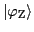solution inV. In this case, the parent and daughter nuclei are axial, which allows us to reduce the angular-momentum projection to one-dimension and extend the basis size up to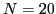HO shells.

With increasing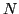,increases, and asymptotically it reaches the value of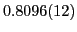%. This limiting value is about 6.7% larger than the value of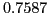% obtained for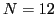shells, that is, for a basis used to compute the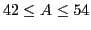cases. For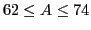nuclei, which were all found to be triaxial, we have used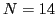shells. The further increase of basis size is practically impossible. Nonetheless, as seen in Fig. 5, a rate of increase ofslows down exponentially with, which supports our 10% error estimate due to the basis truncation.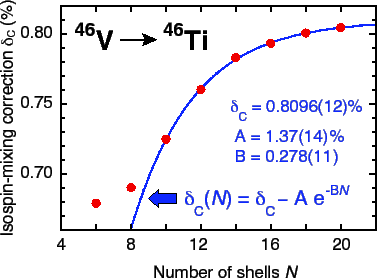The total error of the calculated value ofincludes the standard deviation from the averaging,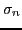, and the assumed 10% uncertainty due to the basis size:. The same prescription for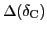was also used in the test calculations with SHZ2, even though a slightly smaller HO basis was employed in that case.

For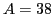nuclei, our model predicts the unusually large correction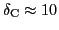%. The origin of a very different isospin mixing obtained for odd-odd and even-even members of this isobaric triplet is not fully understood. Most likely, it is a consequence of the poor spectroscopic properties of SV. Indeed, as a result of an incorrect balance between the spin-orbit and tensor terms in SV, the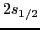subshell is shifted up in energy close to the Fermi surface. This state is more sensitive to time-odd polarizations than other s.p. states around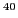Ca core, see Table I in Ref. . The calculated equilibrium deformations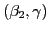of the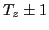and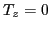isobaric triplet are very similar, around (0.090, 60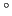). In the following, the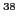KAr transition is excluded from the calculation of the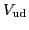matrix element.Next: The survey of ISB Up: ISB corrections to the Previous: Nearly degenerate -orbitals
Jacek Dobaczewski 2012-10-19